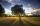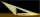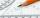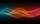The shadow of the tree is 16 meters long. Shadow of two meters high tourist sign beside standing is 3.2 meters long. What height has tree (in meters)?

Result

x =  10 m

Solution:Leave us a comment of example and its solution (i.e. if it is still somewhat unclear...):Be the first to comment!Next similar examples:Nine meters height poplar tree has a shadow 16.2 meters long. How long shadow have at the same time Joe if he is 1,4m tall?A meter pole perpendicular to the ground throws a shadow of 40 cm long, the house throws a shadow 6 meters long. What is the height of the house?
3. TreesAlong the road were planted 250 trees of two types. Cherry for 60 CZK apiece and apple 50 CZK apiece. The entire plantation cost 12,800 CZK. How many was cherries and apples?
4. Liters od milkThe cylinder-shaped container contains 80 liters of milk. Milk level is 45 cm. How much milk will in the container, if level raise to height 72 cm?
5. DonutsFind how many donuts each student will receive if you share 126 donuts in a ratio of 1:5:8
6. MedianThe median of the triangle LMN is away from vertex N 84 cm. Calculate the length of the median, which start at N.
7. Center traverseIt is true that the middle traverse bisects the triangle?If Petra read 10 pages per day, she would read the book two days earlier than she read 6 pages a day. How many pages does a book have?
9. Sides od triangleSides of the triangle ABC has length 4 cm, 5 cm and 7 cm. Construct triangle A'B'C' that are similar to triangle ABC which has a circumference of 12 cm.
10. Smallest internal angleCalculate what size has the smallest internal angle of the triangle if values of angles α:β:γ = 3:4:8
11. Hotel roomsIn the 45 rooms, there were 169 guests, some rooms were three-bedrooms and some five-bedrooms. How many rooms were?
12. MushroomsEva and Jane collected 114 mushrooms together. Eve found twice as much as Jane. How many mushrooms found each of them?
13. EquationsSolve following system of equations: 6(x+7)+4(y-5)=12 2(x+y)-3(-2x+4y)=-44Calculate how many percent will increase the length of an HTML document, if any ASCII character unnecessarily encoded as hexadecimal HTML entity composed of six characters (ampersand, grid #, x, two hex digits and the semicolon). Ie. space as: &#x20;Solve system of linear equations: x-2y=6 3x+2y=4The sum of the three numbers is 287. These numbers are in a ratio of 3: 7: 1/4 Define these numbers52 is what percent of 93?